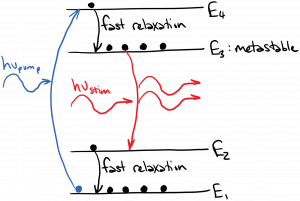# Pumping Schemes

As we saw from Beer’s Law, in order to have optical amplification occur, we must have the density of atoms in the upper energy state$N_2 > (g_2 / g_1) N_1$, or if the degeneracies are equal to one, just$N_2 > N_1$.  As previously mentioned, this is called inversion.  Inversion requires pumping – an injection of energy into the atoms.  This pumping could take many forms.  In gas lasers, an electrical discharge is a common pumping mechanism.  It is also possible to pump with light (optical absorption); however, if we are pumping with light, there must be at least one more atomic energy state involved besides$E_1$ and$E_2$ to make it work.  Why?

Imagine we have a collection of atoms which are all initially in the lower energy state$E_1$.  For simplicity, take$g_2 = g_1 = 1$.  Let’s try to pump atoms into the upper energy state$E_2$ using a light source whose frequency$\nu = (E_2 - E_1) / h$.  This will work fine initially – all the incoming light will be absorbed by the atoms in state 1, and those atoms will be promoted to state 2.  However, as the density of atoms in state 2$N_2$ climbs, eventually we will reach a point where$N_2 = N_1$.  At this point, an incoming photon is equally likely to be absorbed or cause stimulated emission.  Therefore, the light won’t be able to push$N_2$ any higher, at least in steady state.  If we briefly manage to get$N_2 > N_1$, stimulated emission will dominate over absorption, and$N_2$ will be driven back down.

So, we must introduce a third energy state$E_3$ into our consideration.  We could pump atoms from$E_1$ to$E_3$, as shown in the diagram below.  We would be wise to choose a set of states for which there is a fast relaxation process that quickly drops atoms from state$E_3$ to$E_2$.  In this way,$N_3$ will always be close to zero, and pump photons whose frequency$\nu_{pump} = (E_3 - E_1) / h$ will always be absorbed.  At the same time, we would like atoms to linger in state$E_2$ a long time (a so-called ‘metastable’ state).  If we satisfy these conditions, we can increase$N_2$ until it is greater than$N_1$ using the pump from$E_1$ to$E_3$.  Once$N_2 > N_1$, we’ve achieved inversion and therefore amplification for photons whose frequency$\nu_{stim} = (E_2 - E_1) / h$.The three-level scheme above has a weakness.  It is relatively easy to increase$N_2$, but we would also like to decrease$N_1$, in order to maximize the inversion$(N_2 - N_1)$.  If the state$E_1$ is the ground state, the pump rate must be extremely large to lower$N_1$ significantly (a very intense pump would be required).  One way to address this challenge is to introduce a fourth state into the pumping scheme, as diagrammed below.  In this case, we want a pump tuned to the transition from$E_1$ to$E_4$, a fast relaxation process from$E_4$ to$E_3$, a fast relaxation process from$E_2$ to$E_1$, and a metastable state$E_3$.  This scheme is designed to amplify light with frequency$\nu_{stim} = (E_3 - E_2) / h$.  Atoms are pumped up to$E_4$ and quickly drop to$E_3$, where they linger.  After a stimulated emission event drops the atom to state$E_2$, it quickly relaxes to state$E_1$, ensuring that$N_2$ will remain small.   In this way, we can maximize the inversion$(N_3 - N_2)$.Of course, in engineering as in life, no improvement comes without a price.  The 3- and 4-level pumping schemes make it easier to achieve a large inversion.  The price we pay is an inherent loss of efficiency.  If we are pumping with a single photon of energy$h \nu_{pump} = (E_4 - E_1)$, at best we will get out a single photon of energy$h \nu_{stim} = (E_3 - E_2)$.  The energy difference$(h \nu_{pump} - h \nu_{stim})$ represents unavoidable energy loss in this system.